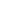# A discount series of 10% , 20% and 40% is equal to a single discount of:

a) 50%
b) 56.8%
c) 60%
d) 60.28%
SSC CHSL-2015

 56.8. Solution First discount is 10% and 2nd discount is 20% then , Total discount of first two discounts = ( 10 + 20 - 10 x 20 / 100 ) % = ( 30 – 200 /100 ) % = 28% Third discount = 40% Total discount = ( 28 + 40 - 28 x 40 /100 )% = 56.8%
Share :
+
Next
« Prev
Prev
Next »